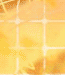## mouse movement question

##### mouse movement question
 Author Message peterposted 9 Months Ago ANSWERGroup: Forum Members Posts: 47, Visits: 171 / ontrialbegin = [picture.bord.hposition = 1px * mouse.x; picture.bord.vposition = 1px * mouse.y]When the trial loops internally across multiple iterations the line of code above fixes the displayed image (picture.board) to the movement of the mouse. Meaning, for example, that as the mouse is moved towards the bottom right, the picture.bord moves with it towards the bottom right. I would like to reverse these relationships so that as you move the mouse towards the bottom right the image (picture.bord) moves towards the top left. I would be very grateful for any assistance in cracking this.Many thanks Peter Daveposted 9 Months Ago ANSWERGroup: Administrators Posts: 12K, Visits: 84K +x peter - 11/2/2021/ ontrialbegin = [picture.bord.hposition = 1px * mouse.x; picture.bord.vposition = 1px * mouse.y]When the trial loops internally across multiple iterations the line of code above fixes the displayed image (picture.board) to the movement of the mouse. Meaning, for example, that as the mouse is moved towards the bottom right, the picture.bord moves with it towards the bottom right. I would like to reverse these relationships so that as you move the mouse towards the bottom right the image (picture.bord) moves towards the top left. I would be very grateful for any assistance in cracking this.Many thanks PeterHere's an ancient example ( https://www.millisecond.com/forums/FindPost4421.aspx ), code slightly cleaned up and adapted to Inquisit 6 syntax./ inputdevice = mouse/ screencolor = (white)/ fontstyle = ("Verdana", 3.00%, true)/ txcolor = (black)/ txbgcolor = (white)/ x = 0px/ y = 0px/ mirrorx = true // mirror horizontally/ mirrory = true // mirror vertically/ x = 1px*abs(values.x-(values.mirrorx*display.canvaswidth))/ y = 1px*abs(values.y-(values.mirrory*display.canvasheight))/ blocks = [1=example]/ trials = [1=start]/ stimulusframes = [1=clickhere]/ validresponse = (lbuttondown)/ ontrialend = [values.x=trial.start.responsex; values.y=trial.start.responsey]/ branch = [trial.move]/ recorddata = false/ ontrialend = [values.x=trial.move.responsex;     values.y=trial.move.responsey;]/ stimulusframes = [1=clearscreen, dot, coordinates]/ validresponse = (mousemove, lbuttondown, rbuttondown)/ numframes = 1/ branch = [if (trial.move.response=="lbuttondown") {        return trial.end;    } else if (trial.move.response == "rbuttondown") {        return trial.pause;    } else if (trial.move.response == "mousemove") {        trial.move;    };]/ recorddata = false/ stimulusframes = [1=coordinates]/ validresponse = (mousemove, lbuttondown)/ numframes = 1/ ontrialend = [values.x=trial.pause.responsex; values.y=trial.pause.responsey]/ branch = [if (trial.pause.response=="lbuttondown") {        return trial.move;    } else {        return trial.pause;    };]/ recorddata = false/ stimulusframes = [1=clearscreen, goodbye]/ validresponse = (lbuttondown)/ recorddata = true/ shape = circle/ width = 4%/ color = (blue)/ erase = false/ hposition = expressions.x/ vposition = expressions.y/ items = ("x=<%values.x%> | y=<%values.y%>")/ position = (50%, 5%)/ size = (27.5%, 4%)/ vjustify = center/ erase = false/ items = ("Click to start.")/ items = ("Goodbye!") peterposted 9 Months Ago ANSWERGroup: Forum Members Posts: 47, Visits: 171 +x Dave - 11/2/2021+x peter - 11/2/2021/ ontrialbegin = [picture.bord.hposition = 1px * mouse.x; picture.bord.vposition = 1px * mouse.y]When the trial loops internally across multiple iterations the line of code above fixes the displayed image (picture.board) to the movement of the mouse. Meaning, for example, that as the mouse is moved towards the bottom right, the picture.bord moves with it towards the bottom right. I would like to reverse these relationships so that as you move the mouse towards the bottom right the image (picture.bord) moves towards the top left. I would be very grateful for any assistance in cracking this.Many thanks PeterHere's an ancient example ( https://www.millisecond.com/forums/FindPost4421.aspx ), code slightly cleaned up and adapted to Inquisit 6 syntax./ inputdevice = mouse/ screencolor = (white)/ fontstyle = ("Verdana", 3.00%, true)/ txcolor = (black)/ txbgcolor = (white)/ x = 0px/ y = 0px/ mirrorx = true // mirror horizontally/ mirrory = true // mirror vertically/ x = 1px*abs(values.x-(values.mirrorx*display.canvaswidth))/ y = 1px*abs(values.y-(values.mirrory*display.canvasheight))/ blocks = [1=example]/ trials = [1=start]/ stimulusframes = [1=clickhere]/ validresponse = (lbuttondown)/ ontrialend = [values.x=trial.start.responsex; values.y=trial.start.responsey]/ branch = [trial.move]/ recorddata = false/ ontrialend = [values.x=trial.move.responsex;     values.y=trial.move.responsey;]/ stimulusframes = [1=clearscreen, dot, coordinates]/ validresponse = (mousemove, lbuttondown, rbuttondown)/ numframes = 1/ branch = [if (trial.move.response=="lbuttondown") {        return trial.end;    } else if (trial.move.response == "rbuttondown") {        return trial.pause;    } else if (trial.move.response == "mousemove") {        trial.move;    };]/ recorddata = false/ stimulusframes = [1=coordinates]/ validresponse = (mousemove, lbuttondown)/ numframes = 1/ ontrialend = [values.x=trial.pause.responsex; values.y=trial.pause.responsey]/ branch = [if (trial.pause.response=="lbuttondown") {        return trial.move;    } else {        return trial.pause;    };]/ recorddata = false/ stimulusframes = [1=clearscreen, goodbye]/ validresponse = (lbuttondown)/ recorddata = true/ shape = circle/ width = 4%/ color = (blue)/ erase = false/ hposition = expressions.x/ vposition = expressions.y/ items = ("x=<%values.x%> | y=<%values.y%>")/ position = (50%, 5%)/ size = (27.5%, 4%)/ vjustify = center/ erase = false/ items = ("Click to start.")/ items = ("Goodbye!")Thank you for that, i will take a look and try to pick out what i need.regardsPeter

#### Merge Selected

Merge into selected topic...

Merge into merge target...

Merge into a specific topic ID...